# Doris Join optimization principle

Doris supports two physical operators, one is Hash Join, and the other is Nest Loop Join.

• Hash Join: Create a hash table on the right table based on the equivalent join column, and the left table uses the hash table to perform join calculations in a streaming manner. Its limitation is that it can only be applied to equivalent joins.
• Nest Loop Join: With two for loops, it is very intuitive. Then it is applicable to unequal-valued joins, such as: greater than or less than or the need to find a Cartesian product. It is a general join operator, but has poor performance.

As a distributed MPP database, data shuffle needs to be performed during the Join process. Data needs to be split and scheduled to ensure that the final Join result is correct. As a simple example, assume that the relationship S and R are joined, and N represents the number of nodes participating in the join calculation; T represents the number of tuples in the relationship.

## Doris Shuffle way​

1. Doris supports 4 Shuffle methods

It requires the full data of the right table to be sent to the left table, that is, each node participating in Join has the full data of the right table, that is, T(R).

Its applicable scenarios are more general, and it can support Hash Join and Nest loop Join at the same time, and its network overhead is N * T(R).The data in the left table is not moved, and the data in the right table is sent to the scanning node of the data in the left table.

2. Shuffle Join

When Hash Join is performed, the corresponding Hash value can be calculated through the Join column, and Hash bucketing can be performed.

Its network overhead is: T(S) + T(R), but it can only support Hash Join, because it also calculates buckets according to the conditions of Join.The left and right table data are sent to different partition nodes according to the partition, and the calculated demerits are sent.

3. Bucket Shuffle Join

Doris's table data itself is bucketed by Hash calculation, so you can use the properties of the bucketed columns of the table itself to shuffle the Join data. If two tables need to be joined, and the Join column is the bucket column of the left table, then the data in the left table can actually be calculated by sending the data into the buckets of the left table without moving the data in the right table.

Its network overhead is: T(R) is equivalent to only Shuffle the data in the right table.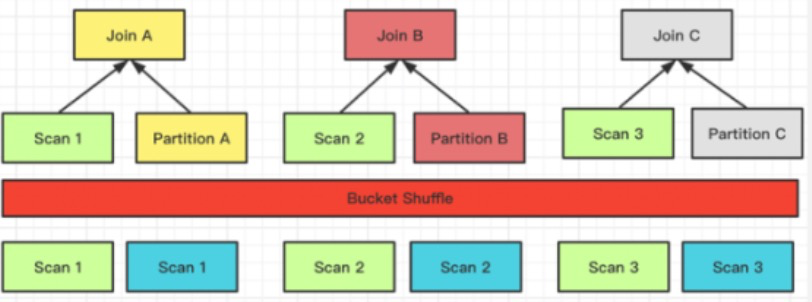The data in the left table does not move, and the data in the right table is sent to the node that scans the table in the left table according to the result of the partition calculation.

4. Colocation

It is similar to Bucket Shuffle Join, which means that the data has been shuffled according to the preset Join column scenario when data is imported. Then the join calculation can be performed directly without considering the Shuffle problem of the data during the actual query.The data has been pre-partitioned, and the Join calculation is performed directly locally

### Comparison of four Shuffle methods​

BroadCastN * T(R)Hash Join / Nest Loop JoinUniversal
ShuffleT(S) + T(R)Hash JoinGeneral
Bucket ShuffleT(R)Hash JoinThere are distributed columns in the left table in the join condition, and the left table is executed as a single partition
Colocate0Hash JoinThere are distributed columns in the left table in the join condition, and the left and right tables belong to the same Colocate Group

N : The number of Instances participating in the Join calculation

T(relation) : Tuple number of relation

The flexibility of the above four methods is from high to low, and its requirements for this data distribution are becoming more and more strict, but the performance of Join calculation is also getting better and better.

## Runtime Filter Join optimization​

Doris will build a hash table in the right table when performing Hash Join calculation, and the left table will stream through the hash table of the right table to obtain the join result. The RuntimeFilter makes full use of the Hash table of the right table. When the right table generates a hash table, a filter condition based on the hash table data is generated at the same time, and then pushed down to the data scanning node of the left table. In this way, Doris can perform data filtering at runtime.

If the left table is a large table and the right table is a small table, then using the filter conditions generated by the left table, most of the data to be filtered in the Join layer can be filtered in advance when the data is read, so that a large amount of data can be filtered. Improve the performance of join queries.

Currently Doris supports three types of RuntimeFilter

• One is IN-IN, which is well understood, and pushes a hashset down to the data scanning node.
• The second is BloomFilter, which uses the data of the hash table to construct a BloomFilter, and then pushes the BloomFilter down to the scanning node that queries the data. .
• The last one is MinMax, which is a Range range. After the Range range is determined by the data in the right table, it is pushed down to the data scanning node.

There are two requirements for the applicable scenarios of Runtime Filter:

• The first requirement is that the right table is large and the left table is small, because building a Runtime Filter needs to bear the computational cost, including some memory overhead.
• The second requirement is that there are few results from the join of the left and right tables, indicating that this join can filter out most of the data in the left table.

When the above two conditions are met, turning on the Runtime Filter can achieve better results

When the Join column is the Key column of the left table, the RuntimeFilter will be pushed down to the storage engine. Doris itself supports delayed materialization,

Delayed materialization is simply like this: if you need to scan three columns A, B, and C, there is a filter condition on column A: A is equal to 2, if you want to scan 100 rows, you can scan 100 rows of column A first, Then filter through the filter condition A = 2. After filtering the results, read columns B and C, which can greatly reduce the data read IO. Therefore, if the Runtime Filter is generated on the Key column, and the delayed materialization of Doris itself is used to further improve the performance of the query.

### Runtime Filter Type​

• Doris provides three different Runtime Filter types:
• The advantage of IN is that the effect filtering effect is obvious and fast. Its shortcomings are: First, it only applies to BroadCast. Second, when the right table exceeds a certain amount of data, it will fail. The current Doris configuration is 1024, that is, if the right table is larger than 1024, the Runtime Filter of IN will directly failed.
• The advantage of MinMax is that the overhead is relatively small. Its disadvantage is that it has a relatively good effect on numeric columns, but basically no effect on non-numeric columns.
• The feature of Bloom Filter is that it is universal, suitable for various types, and the effect is better. The disadvantage is that its configuration is more complicated and the calculation is high.

Once the database involves multi-table Join, the order of Join has a great impact on the performance of the entire Join query. Assuming that there are three tables to join, refer to the following picture, the left is the a table and the b table to do the join first, the intermediate result has 2000 rows, and then the c table is joined.

Next, look at the picture on the right and adjust the order of Join. Join the a table with the c table first, the intermediate result generated is only 100, and then finally join with the b table for calculation. The final join result is the same, but the intermediate result it generates has a 20x difference, which results in a big performance diff.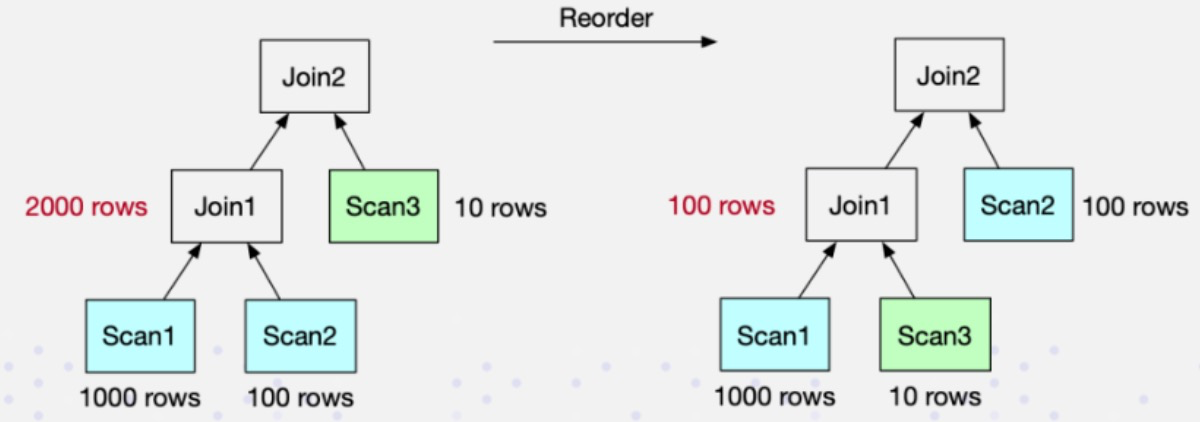• Doris currently supports the rule-based Join Reorder algorithm. Its logic is:
• Make joins with large tables and small tables as much as possible, and the intermediate results it generates are as small as possible.
• Put the conditional join table forward, that is to say, try to filter the conditional join table
• Hash Join has higher priority than Nest Loop Join, because Hash Join itself is much faster than Nest Loop Join.

## Doris Join optimization method​

Doris Join tuning method:

• Use the Profile provided by Doris itself to locate the bottleneck of the query. Profile records various information in Doris' entire query, which is first-hand information for performance tuning. .
• Understand the Join mechanism of Doris, which is also the content shared with you in the second part. Only by knowing why and understanding its mechanism can we analyze why it is slow.
• Use Session variables to change some behaviors of Join, so as to realize the tuning of Join.
• Check the Query Plan to analyze whether this tuning is effective.

The above 4 steps basically complete a standard Join tuning process, and then it is to actually query and verify it to see what the effect is.

If the first 4 methods are connected in series, it still does not work. At this time, it may be necessary to rewrite the Join statement, or to adjust the data distribution. It is necessary to recheck whether the entire data distribution is reasonable, including querying the Join statement, and some manual adjustments may be required. Of course, this method has a relatively high mental cost, which means that further analysis is required only when the previous method does not work.

## Optimization case practice​

### Case 1​

A four-table join query, through Profile, found that the second join took a long time, taking 14 seconds.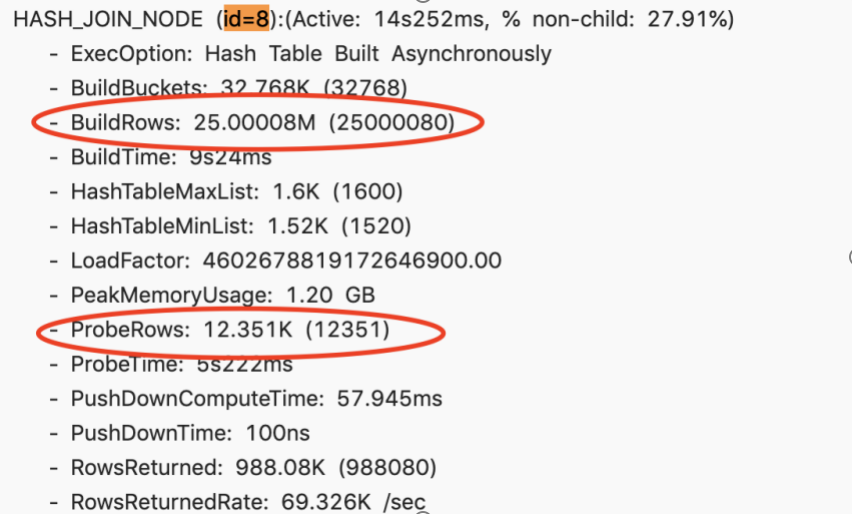After further analysis of Profile, it is found that BuildRows, that is, the data volume of the right table is about 25 million. And ProbeRows (ProbeRows is the amount of data in the left table) is only more than 10,000. In this scenario, the right table is much larger than the left table, which is obviously an unreasonable situation. This obviously shows that there is some problem with the order of Join. At this time, try to change the Session variable and enable Join Reorder.

``set enable_cost_based_join_reorder = true``

This time, the time has been reduced from 14 seconds to 4 seconds, and the performance has been improved by more than 3 times.

At this time, when checking the profile again, the order of the left and right tables has been adjusted correctly, that is, the right table is a large table, and the left table is a small table. The overhead of building a hash table based on a small table is very small. This is a typical scenario of using Join Reorder to improve Join performance.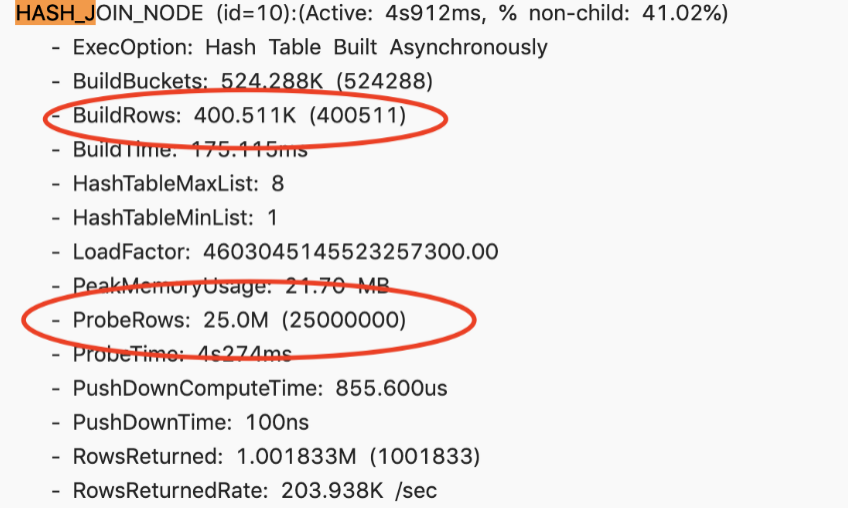### Case 2​

There is a slow query. After viewing the Profile, the entire Join node takes about 44 seconds. Its right table has 10 million, the left table has 60 million, and the final returned result is only 60 million.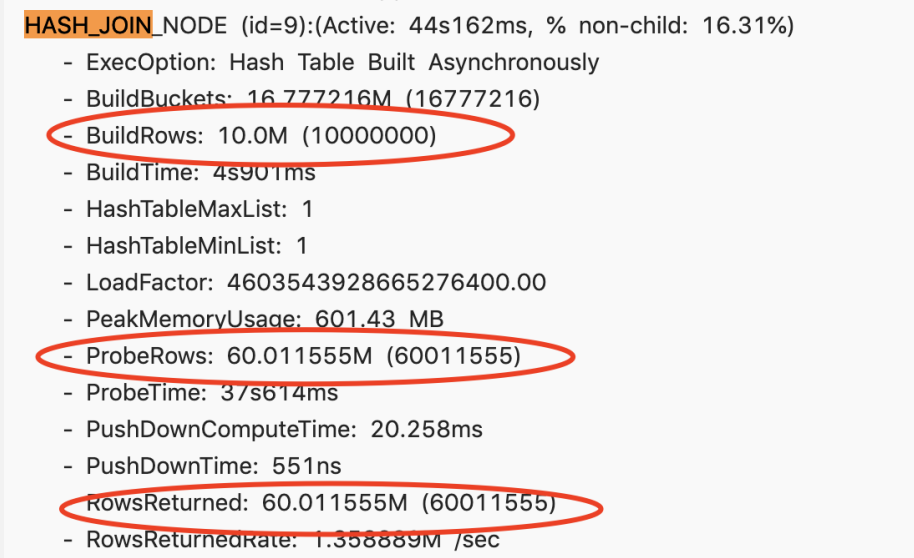It can be roughly estimated that the filtering rate is very high, so why does the Runtime Filter not take effect? Check it out through Query Plan and find that it only enables the Runtime Filter of IN.When the right table exceeds 1024 rows, IN will not take effect, so there is no filtering effect at all, so try to adjust the type of RuntimeFilter.

This is changed to BloomFilter, and the 60 million pieces of data in the left table have filtered 59 million pieces. Basically, 99% of the data is filtered out, and this effect is very significant. The query has also dropped from the original 44 seconds to 13 seconds, and the performance has been improved by about three times.

### Case 3​

The following is a relatively extreme case, which cannot be solved by tuning some environment variables, because it involves SQL Rewrite, so the original SQL is listed here.

``select 100.00 * sum (case        when P_type like 'PROMOS'        then 1 extendedprice * (1 - 1 discount)        else 0        end ) / sum(1 extendedprice * (1 - 1 discount)) as promo revenuefrom lineitem, partwhere    1_partkey = p_partkey    and 1_shipdate >= date '1997-06-01'    and 1 shipdate < date '1997-06-01' + interval '1' month``

This Join query is very simple, a simple join of left and right tables. Of course, there are some filter conditions on it. When I opened the Profile, I found that the entire query Hash Join was executed for more than three minutes. It is a BroadCast Join, and its right table has 200 million entries, while the left table has only 700,000. In this case, it is unreasonable to choose Broadcast Join, which is equivalent to making a Hash Table of 200 million records, and then traversing the Hash Table of 200 million records with 700,000 records, which is obviously unreasonable.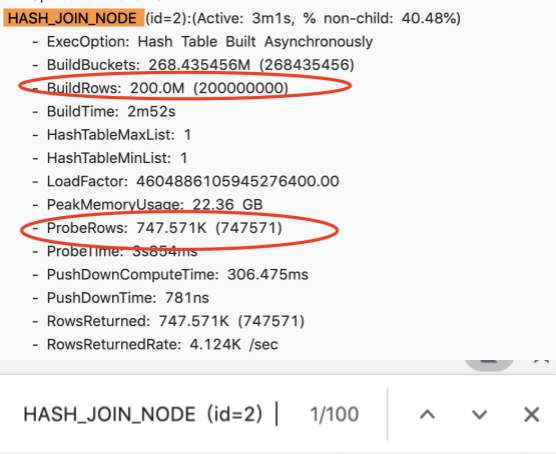Why is there an unreasonable Join order? In fact, the left table is a large table with a level of 1 billion records. Two filter conditions are added to it. After adding these two filter conditions, there are 700,000 records of 1 billion records. But Doris currently does not have a good framework for collecting statistics, so it does not know what the filtering rate of this filter condition is. Therefore, when the join order is arranged, the wrong left and right table order of the join is selected, resulting in extremely low performance.

The following figure is an SQL statement after the rewrite is completed. A Join Hint is added after the Join, a square bracket is added after the Join, and then the required Join method is written. Here, Shuffle Join is selected. You can see in the actual query plan on the right that the data is indeed Partitioned. The original 3-minute time-consuming is only 7 seconds after the rewriting, and the performance is improved significantly.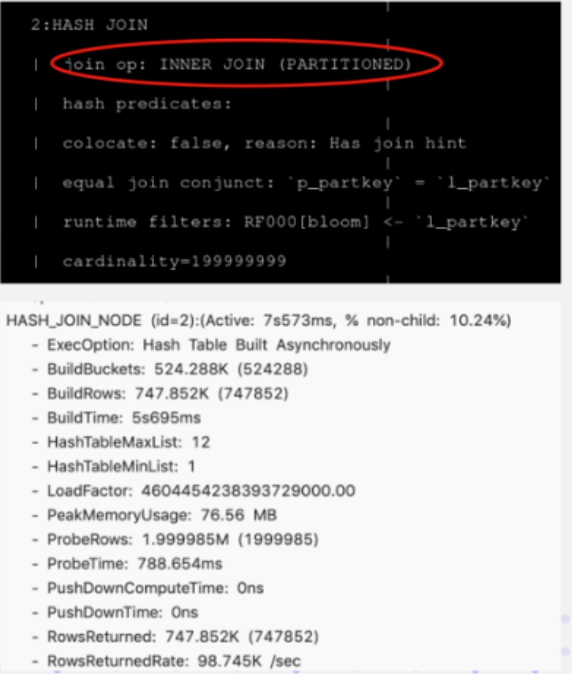## Doris Join optimization suggestion​

Finally, we summarize four suggestions for optimization and tuning of Doris Join:

• The first point: When doing Join, try to select columns of the same type or simple type. If the same type is used, reduce its data cast, and the simple type itself joins the calculation quickly.
• The second point: try to choose the Key column for Join. The reason is also introduced in the Runtime Filter. The Key column can play a better effect on delayed materialization.
• The third point: Join between large tables, try to make it Co-location, because the network overhead between large tables is very large, if you need to do Shuffle, the cost is very high.
• Fourth point: Use Runtime Filter reasonably, which is very effective in scenarios with high join filtering rate. But it is not a panacea, but has certain side effects, so it needs to be switched according to the granularity of specific SQL.
• Finally: When it comes to multi-table Join, it is necessary to judge the rationality of Join. Try to ensure that the left table is a large table and the right table is a small table, and then Hash Join will be better than Nest Loop Join. If necessary, you can use SQL Rewrite to adjust the order of Join using Hint.